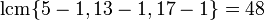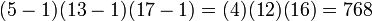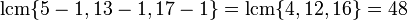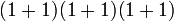# 1105

## Summary

### Factorization

1105 is a square-free number. It has prime factors 5, 13, and 17:### Properties and families

Property or family Parameter values First few numbers satisfying the property Proof of satisfaction/containment/membership
Carmichael number (also called absolute pseudoprime) second Carmichael number 561, 1105, 1729, 2465, 2821, 6601, [SHOW MORE]View list on OEIS The universal exponent iswhich divides 1105 - 1 = 1104.
Poulet number (also called Sarrus number) (Fermat pseudoprime to base 2) fourth Poulet number 341, 561, 645, 1105, 1387, 1729, 1905, 2047, [SHOW MORE] View list on OEIS follows from its being a Carmichael number (note that Carmichael number is odd).

## Arithmetic functions

Function Value Explanation
Euler totient function 768 The Euler totient function is.
universal exponent 48 The universal exponent is.
Mobius function -1 The number is a square-free number and it has an odd number of prime divisors (3 prime divisors)
divisor count function 8where the first 1s in each sum denote the multiplicities of the prime divisors.
largest prime divisor 17
largest prime power divisor 17